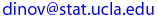Ivo Dinov
UCLA Statistics, Neurology, LONI
 Courses SOCR Ivo Dinov's Home SiteMap Software Contact

##### Introduction to Statistical Methods for the Life and Health Sciences

Textbook & Syllabus

Textbook:
Chance Encounters - A first Course in Data Analysis and Inference by Christopher Wild and George Seber (2000)
Tentative schedule of topics to be covered
1. Data Collection, Experiments, Observational Studies vs. Designed Experiments, Ch. 1
2. Variables Graphical & Numerical Summaries, Ch. 2
3. Probability & Independence, Ch. 4
4. Discrete Random Variables, Binomial Distribution, Expected Value, Variance, Ch. 5
5. Bayes' Rule, supplement
6. Continuous Random Variables, Normal Distribution, Ch. 6
7. Inference, Means, Central Limit Theorem, Ch. 7
8. Confidence Intervals, Ch. 8
9. Hypothesis Testing, Ch. 9
10. Z-test for mean and proportion, Ch. 10
11. 2 independent samples, t-test, Ch. 7, 8, 9, 10
12. Bivariate Data, Correlation, Ch. 3
13. Regression, Ch. 12
14. ANOVA, Chi-square, Ch. 11

 Ivo Dinov's Home http://www.stat.ucla.edu/~dinov Visitor number, since Jan. 01, 2002 © I.D.Dinov 1997-2003
Last modified on by